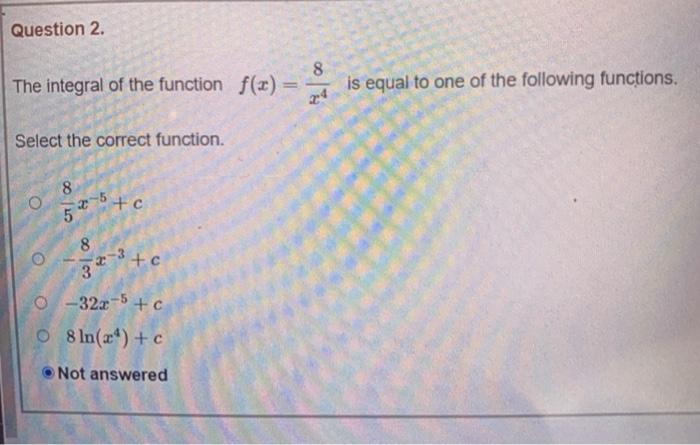### Create an Account

Already have account?

### Forgot Your Password ?

Home / Questions / Question 2. 8 The integral of the function f(x) is equal to one of the following functions...

# Question 2. 8 The integral of the function f(x) is equal to one of the following functions. Select the correct function. 8 5 x 5 to O 8 2-3+c 3 0-32x-5 + c O 8 ln() + c Not answered

Question 2. 8 The integral of the function f(x) is equal to one of the following functions. Select the correct function. 8 5 x 5 to O 8 2-3+c 3 0-32x-5 + c O 8 ln() + c Not answeredApr 25 2021 View more View Less

#### Answer (Solved)Subscribe To Get Solution